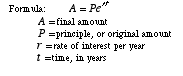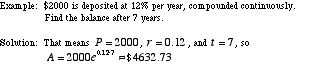index: click on a letter A B C D E F G H I J K L M N O P Q R S T U V W X Y Z A to Z index index: subject areas numbers & symbols sets, logic, proofs geometry algebra trigonometry advanced algebra & pre-calculus calculus advanced topics probability & statistics real world applications multimedia entrieswww.mathwords.com about mathwords website feedback

 Continuously Compounded Interest Interest that is, hypothetically, computed and added to the balance of an account every instant. This is not actually possible, but continuous compounding is well-defined nevertheless as the upper bound of "regular" compound interest. The formula, given below, is sometimes called the shampoo formula (Pert®). Note: This same formula can be used for exponential growth and exponential decay.See also